Previous Page | Next Page

 Working with Matrices

## Subscript Reduction Operators

You can use reduction operators, which return a matrix of reduced dimension, in place of values for subscripts to get reductions across all rows and columns. Table 4.3 lists the eight operators for subscript reduction in IML.

Table 4.3: Subscript Reduction Operators
 Operator Action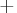additionmultiplication > maximum < minimumindex of maximum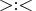index of minimummeansum of squares

For example, to get column sums of the matrix(sum across the rows, which reduces the row dimension to 1), specify X
. The first subscript (+) specifies that summation reduction take place across the rows. Omitting the second subscript, corresponding to columns, leaves the column dimension unchanged. The elements in each column are added, and the new matrix consists of one row containing the column sums.

You can use these operators to reduce either rows or columns or both. When both rows and columns are reduced, row reduction is done first.

For example, the expression Aresults in the maximum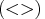of the column sums.

You can repeat reduction operators. To get the sum of the row maxima, use the expression A.

A subscript such as Afirst selects the second and third rows of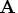and then finds the row sums of that matrix. The following examples demonstrate how to use the operators for subscript reduction.

Consider the following matrix:
The following statements are true: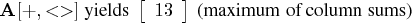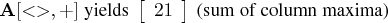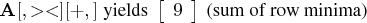Previous Page | Next Page | Top of Page